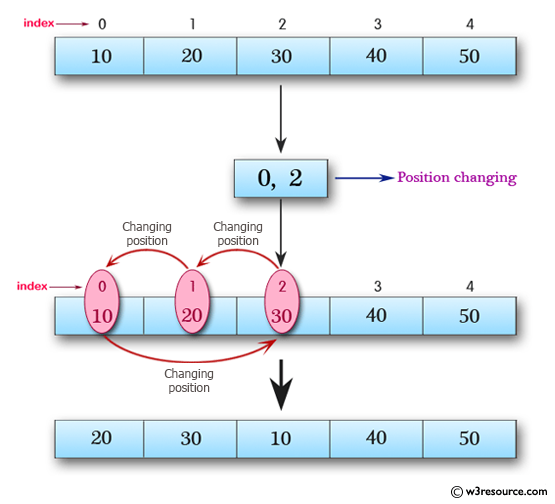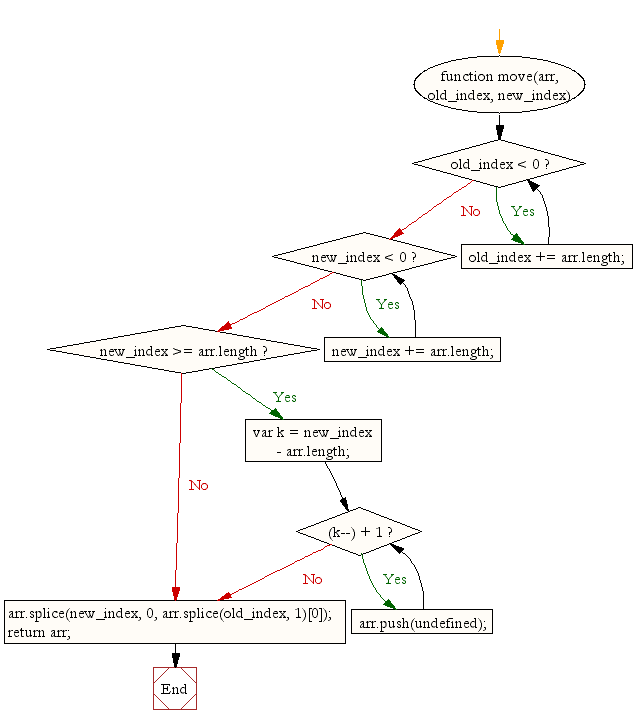# JavaScript: Move an array element from one position to another

## JavaScript Array: Exercise-38 with Solution

Write a JavaScript function to move an array element from one position to another.

Test Data:
console.log(move([10, 20, 30, 40, 50], 0, 2));
[20, 30, 10, 40, 50]
console.log(move([10, 20, 30, 40, 50], -1, -2));
[10, 20, 30, 50, 40]

Pictorial Presentation:Sample Solution:

HTML Code:

``````<!DOCTYPE html>
<html>
<meta charset="utf-8">
<title>JavaScript function to move an array element from one position to another.</title>
<body>

</body>
</html>
```
```

JavaScript Code:

``````function move(arr, old_index, new_index) {
while (old_index < 0) {
old_index += arr.length;
}
while (new_index < 0) {
new_index += arr.length;
}
if (new_index >= arr.length) {
var k = new_index - arr.length;
while ((k--) + 1) {
arr.push(undefined);
}
}
arr.splice(new_index, 0, arr.splice(old_index, 1));
return arr;
}

console.log(move([10, 20, 30, 40, 50], 0, 2));

console.log(move([10, 20, 30, 40, 50], -1, -2));
```
```

Sample Output:

```[20,30,10,40,50]
[10,20,30,50,40]
```

Flowchart:ES6 Version:

``````function move(arr, old_index, new_index) {
while (old_index < 0) {
old_index += arr.length;
}
while (new_index < 0) {
new_index += arr.length;
}
if (new_index >= arr.length) {
let k = new_index - arr.length;
while ((k--) + 1) {
arr.push(undefined);
}
}
arr.splice(new_index, 0, arr.splice(old_index, 1));
return arr;
}

console.log(move([10, 20, 30, 40, 50], 0, 2));

console.log(move([10, 20, 30, 40, 50], -1, -2));
``````

Live Demo :

See the Pen JavaScript - Move an array element from one position to another-array-ex- 38 by w3resource (@w3resource) on CodePen.

Improve this sample solution and post your code through Disqus

What is the difficulty level of this exercise?

Test your Programming skills with w3resource's quiz.

﻿

## JavaScript: Tips of the Day

Returns true if the given number is a power of 2, false otherwise

Example:

```const tips_isPowerOfTwo = n => !!n && (n & (n - 1)) == 0;
console.log(tips_isPowerOfTwo(0));
console.log(tips_isPowerOfTwo(4));
console.log(tips_isPowerOfTwo(16));
```

Output:

```false
true
true
```